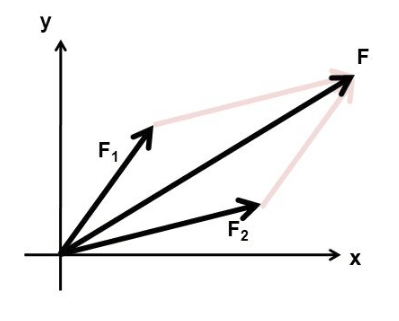# Sum of the two vectors - the result

The sum of two vectors given magnitudes and included angle. Typically two forces.
°Vector addition is the operation of adding two (or more) vectors together into a vector sum. The so-called parallelogram law gives the rule for vector addition of two vectors. For two vectors, the vector sum is obtained by placing them head to tail and drawing the vector from the free tail to the free head.